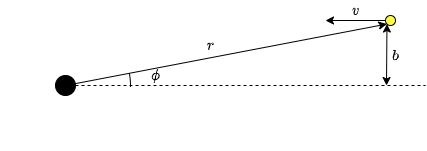# 4.1: Impact Parameter

•• Skidmore College
$$\newcommand{\vecs}{\overset { \rightharpoonup} {\mathbf{#1}} }$$ $$\newcommand{\vecd}{\overset{-\!-\!\rightharpoonup}{\vphantom{a}\smash {#1}}}$$$$\newcommand{\id}{\mathrm{id}}$$ $$\newcommand{\Span}{\mathrm{span}}$$ $$\newcommand{\kernel}{\mathrm{null}\,}$$ $$\newcommand{\range}{\mathrm{range}\,}$$ $$\newcommand{\RealPart}{\mathrm{Re}}$$ $$\newcommand{\ImaginaryPart}{\mathrm{Im}}$$ $$\newcommand{\Argument}{\mathrm{Arg}}$$ $$\newcommand{\norm}{\| #1 \|}$$ $$\newcommand{\inner}{\langle #1, #2 \rangle}$$ $$\newcommand{\Span}{\mathrm{span}}$$ $$\newcommand{\id}{\mathrm{id}}$$ $$\newcommand{\Span}{\mathrm{span}}$$ $$\newcommand{\kernel}{\mathrm{null}\,}$$ $$\newcommand{\range}{\mathrm{range}\,}$$ $$\newcommand{\RealPart}{\mathrm{Re}}$$ $$\newcommand{\ImaginaryPart}{\mathrm{Im}}$$ $$\newcommand{\Argument}{\mathrm{Arg}}$$ $$\newcommand{\norm}{\| #1 \|}$$ $$\newcommand{\inner}{\langle #1, #2 \rangle}$$ $$\newcommand{\Span}{\mathrm{span}}$$$$\newcommand{\AA}{\unicode[.8,0]{x212B}}$$

In this chapter we will investigate the paths that photons take in Schwarzschild spacetime. That may seem like a silly question at first since it is often said that light travels in straight lines, but remember that General Relativity messes with the rules of geometry, so photons can travel in what appears to us as a curved path.

In the previous chapter, we learned that the motion of particles in Schwarzschild spacetime can be described using the energy per unit mass,

$\dfrac{E}{m}=\left(1-\dfrac{2M}{r}\right)\dfrac{dt}{d\tau},\nonumber$

and the angular momentum per unit mass,

$\dfrac{L}{m}=r^2\sin^2\theta\dfrac{d\phi}{d\tau}.\nonumber$

These don't make sense applied to a photon because $$m=0$$ and $$d\tau=0$$ for photons, which makes both sides undefined. If we take the ratio of these two quantities, however, then the problematic parts cancel out. Let's define this ratio as $$b$$.

\begin{align*} b&=\dfrac{L/m}{E/m}&& \text{definition} \\[4pt] b&=\dfrac{L}{E}&&\text{cancel out m} \\[4pt] b&=\dfrac{r^2\sin^2\theta\dfrac{d\phi}{d\tau}}{\left(1-\dfrac{2M}{r}\right)\dfrac{dt}{d\tau}}&&\text{substitute}\\[4pt] b&=\dfrac{r^2\sin^2\theta}{1-\dfrac{2M}{r}} \dfrac{d\phi}{dt}&&\text{cancel }d\tau\text{ for final answer}\end{align*}

In principle, if you have a value for $$b$$, then you can determine $$\phi (t)$$. But that raises the question of how you determine $$b$$ if neither $$E/m$$ nor $$L/m$$ is well-defined for a photon. It turns out that $$b$$ is what is commonly referred to in physics at the impact parameter of the photon (see Box 4.1.1).

## Definition: Impact Parameter

The impact parameter of a photon is basically how much it would miss the Schwarzschild center (i.e. the singularity) by if it were to move undeflected. More formally, it is the perpendicular distance between the line of motion and the radial line that is parallel to it when the photon is very far away. See Fig. 4.1.1.Figure $$\PageIndex{1}$$: The impact parameter b is the perpendicular distance between the line of motion and the radial line that is parallel to it when the photon is very far away. (Copyright; author via source)

## Box $$\PageIndex{1}$$

You may recall from introductory mechanics that the magnitude of the angular momentum of a particle can be written as $$L=rp\sin\phi$$ where p is the linear momentum. If you look at the triangle in Fig. 4.1.1, you can see that $$r\sin\phi=b$$. Use these, along with the energy-momentum relationship $$m^2=E^2-p^2$$ to show that $$\dfrac{L}{E}=b$$ for a photon. (Note that this particular energy-momentum relationship only holds in flat spacetime, but that's okay since the stated definition of b is for a photon that is very far away.)